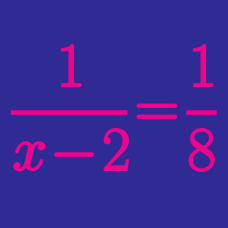Algebra

# Rational Functions - Asymptotes

If the asymptotes of the curve $y=\frac{6x+10}{x+1}$ are $x=a$ and $y=b$, what is the value of $a+b$?

If the graph of $y=\frac{ax-4}{x+b}$ does not intersect either of the lines $x=4$ or $y=-4$, what is the value of $ab$?

If the asymptotes of the curve $y-1=\frac{5x-18}{x-5}$ are $x=a$ and $y=b$, what is the value of $a+b$?

If the asymptotes of the curve $y=-\frac{1}{x-4}+3$ are $x=a$ and $y=b$, what is the value of $a+b$?

If the asymptotes of the curve $y=-\frac{1}{x-4}+11$ are $x=a$ and $y=b$, what is the value of $a+b$?

×